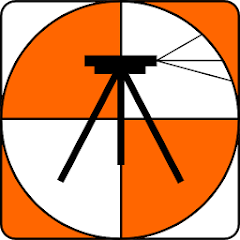# Harcourt Traverse & Surveying

1K+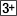Rated for 3+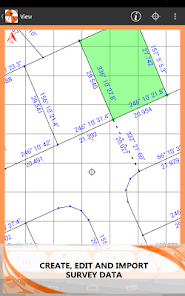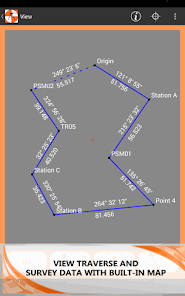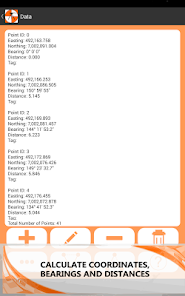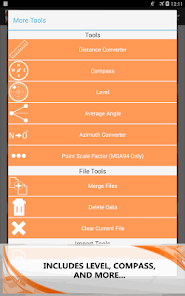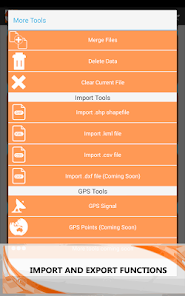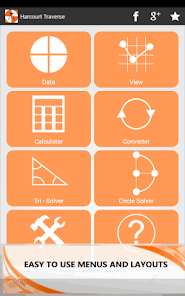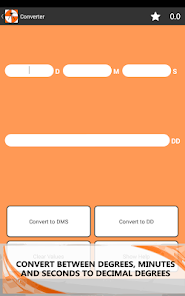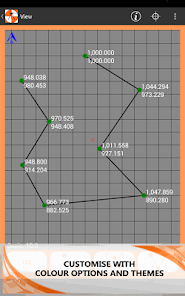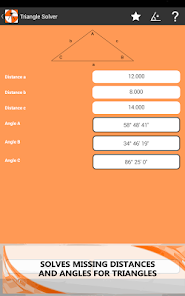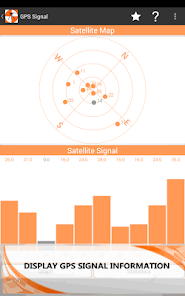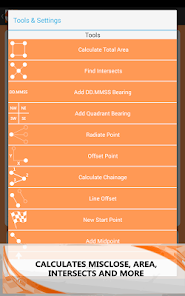Are you a surveyor? Interested in traverse mapping or simply looking for some useful conversion or surveying tools to use at work, at home or in the classroom?

With this app you can quickly calculate and map surveying and traverse data. Also included is a range of surveying calculators such as 3 point circle solver, triangle solver and easy conversions between units of measurement.

With the ability to import surveying data from kml, shp, csv and dxf files you can bring GIS and surveying data to the field for quick checking and calculations.

Useful for surveyors, engineers, students and teachers needing a quick and easy to use traverse and surveying program.

Features Include:
• Calculate coordinates, bearings and distances.
• Large variety of surveying functions and tools.
• Draw points and bearings on a customizable map with the ability to pan and zoom.
• Save and load multiple traverse files.
• Import points, lines and polygons from different file formats such as kml, shp, dxf and csv.
• Includes a range of other practical surveying utilities and math tools.
• Works offline.
• No in app purchases.

Surveying Functions:
• Add, edit and delete points and bearings.
• Calculate bearing and distance between points.
• Find intersect points between bearings.
• Calculate total traverse area.
• Swing bearings by a positive or negative angle.
• Calculate midpoint between two coordinates.
• Calculate chainage along a bearing.
• Radiate from a point using bearing and distance.
• Calculate line and point offset.
• Translate and move coordinates.
• Import GIS data from .kml and .shp files.
• Import points from .csv files.
• Save and load multiple traverse files.
• Export traverse data to CSV, TXT and email files.

Map Functions:
• Pan and zoom
• Display GPS location and GPS accuracy radius.
• Customize map with a range of different colours and options.
• Export map as an image to the photo gallery.

Includes:
• Angle Converter – Convert between decimal degrees (DD) and degrees, minutes and seconds (DMS).
• Angle Calculator – Add or subtract two angles in either DD or DMS.
• Average Angle – Calculate the average between two angles in DMS or DD.
• Azimuth Converter – Converter between azimuth and bearings.
• Compass – Displays orientation in DMS and DD.
• Distance Converter – Convert between commonly used units of measure.
• GPS Signal – Display GPS data and statistics.
• Level – Show the angle and inclination of the device. (Includes spirit level)
• Triangle Solver – Calculate missing angles, distances, area and perimeter.
• 3 Point Circle Solver – Calculate radius, centre point and area of a circle given three points.
Updated on
Mar 22, 2015

## Data safety